## 1.

The Correct Answer is (D) — For this question, you have to group like terms. Multiplying the constants gives –18. Multiplying the variables gives the following:

The result is –18x. If you chose (C), you probably multiplied the exponents rather than adding them.

## 2.

The Correct Answer is (G) — Simply divide the number of particles by 2:

If you chose (F), you probably divided the exponent by 2 as well.

## 3.

The Correct Answer is (E) — You know that (0.20) x = 15, therefore 20% of x is 15. So:

75% of 120 is (120)(0.75) = 90.

## 4.

The Correct Answer is (H) — Recall that distance = speed time. To find the distance that Maria traveled, substitute the values from the question into the formula. Remember to convert the extra 15 minutes into hours by multiplying by the conversion factor for minutes to hours:

Add this to the 5 hours to get a total of 5.25 hours driving, then multiply by speed to find distance:

If you chose (G), you probably didn’t convert the minutes into hours.

## 38.

The Correct Answer is (H) — Use the Pythagorean Theorem and set the height as one of the legs of the triangle, with the cable length acting as the hypotenuse:

\begin{align*} c^2&=a^2+b^2 \\ (13)^2&=5^2+b^2 \\ (13)^3-5^2&=b^2 \\ 144&=b^2 \\ 12&=b \end{align*}

Since a height of -12 ft. is impossible, the height of the tent is 12 ft.
You may have recognized that 13-12-5 is a Pythagorean Triple. If you did, solving this question would have been much easier and quicker.

## 39.

The Correct Answer is (C) — Let x represent Corwin’s favorite number:

\begin{align*} 5&=\sqrt{x+2} \\ 5^2&=x+2 \\ 25-3&=x \\ 23&=x \end{align*}

## 40.

The Correct Answer is (H) — A quick sketch of the circle may help you to solve this question: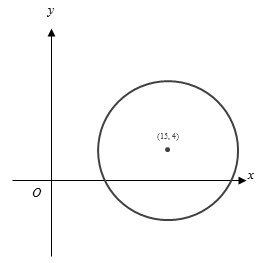Recall the equation of a circle, , where h and k are the center.

You know from the equation that the center is at (15, 4) and that the radius is 9. The circle will not cross the y-axis, since the center is further from the y-axis than the length of the radius. The circle will, however, cross the x-axis because the distance from the center of the circle to the x-axis is smaller than the length of the radius. Since the x-axis is not tangent to the circle, it will intersect the circle twice.

If you chose (G), you may have missed that a circle will intersect the x-axis more than once. If you chose (F), you may have miscalculated the radius or center of the circle and misplaced it on the coordinate plane.

## 41.

The Correct Answer is (B) — When vector is broken up into its components, it is -5 units in the direction of the i unit vector (parallel to the x-axis) and 3 units in the direction of the j unit vector (parallel to the y-axis). Hence, the component form is .

## 42.

The Correct Answer is (F) — In order for to be true, . Therefore, and . Remember that you are not finished yet: .
If you chose (G) or (J), you may have found just the value of x or y without multiplying them together.

## 43.

The Correct Answer is (C) — Since the radius is tripled, sub 3r in for r.
\begin{align*} A&=\frac{4}{3}\pi(r)^3 \\ A&=\frac{4}{3}\pi(3r)^3 \\ A&=\frac{4}{3}\pi(r)^3\times27 \\ \end{align*}

The volume will increase by a factor of 27.

## 44.

The Correct Answer is (G) — Start by finding . Then, substitute into .

## 45.

The Correct Answer is (B) — In order for four points to form a square, all points must be an equal distance from its adjacent points. A quick sketch may help you with this problem: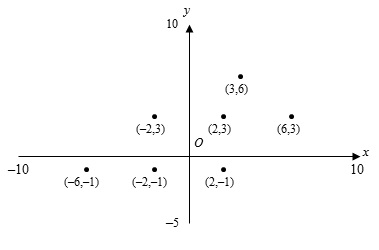Answer choice (A) is from (2, -1) and (2, -1) is from (-2, 3). Answer choice (C) is 4 units away from both provided points. Answer choice (D) is 4 units away from both provided points. Answer choice E is from (2, -1) and (2, -1) is from (-2, 3). Answer choice (B) is from (-2, 3) but (-2, 3) is from (2, -1).

## 46.

The Correct Answer is (F) — Recall that the sum of any quadrilateral’s interior angles is always 360ᣞ. To find the value of x: 360ᣞ — 85ᣞ — 105ᣞ — 70ᣞ = 100ᣞ.

## 47.

The Correct Answer is (A) — To find the length of , subtract the length of from . Then, collect like terms and simplify.

\begin{align*} &=3x+5a-(x-2a) \\ &=3x+5a-x+2a \\ &=2x+7a \end{align*}

## 48.

The Correct Answer is (H) — The question tells you that the triangle is an isosceles right triangle, so you know that both of the legs of the triangle are the same size and that you can use the Pythagorean Theorem.

\begin{align*} (\frac{10}{\sqrt{2}})^2+(\frac{10}{\sqrt{2}})^2&=c^2 \\ \frac{100}{2}+\frac{100}{2}&=c^2 \\ 100&=c^2 \\ c&=10 \end{align*}

## 49.

The Correct Answer is (C) — By looking at the diagram, you can see that the area of the garden with pansies is equivalent to 2/4 of the garden, minus the 2 small squares of lilies.

\begin{align*} &=2(\frac{100}{4})-2(\frac{\frac{100}{4}}{4}) \\ &=2(25)-2(\frac{25}{4}) \\ &=50-12.5 \\ &=37.5 \\ &\approx38 \end{align*}

## 50.

The Correct Answer is (H) — To answer this question, a diagram may help: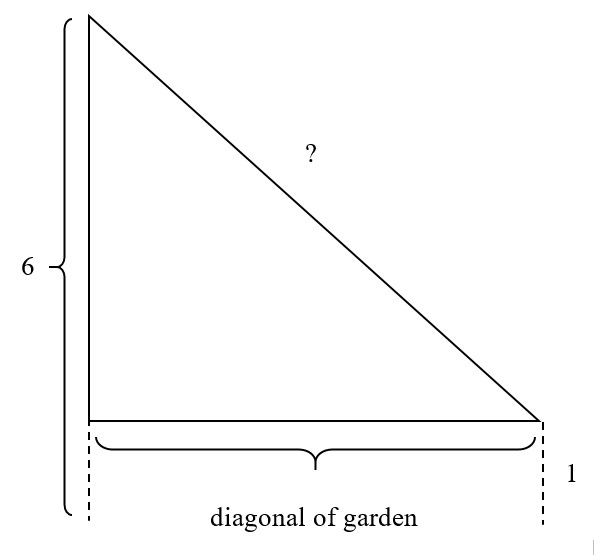Using the Pythagorean Theorem, you can find the diagonal of the garden:

\begin{align*} (10)^2+(10)^2&=d^2 \\ 200&=d^2 \\ d&=\sqrt{200} \\ \end{align*} Now, you can find the length of the clothesline.

\begin{align*} (\sqrt{200})^2+5^2&=c^2 \\ 200+25&=c^2 \\ 225&=c^2 \\ c&=15 \end{align*}

## 51.

The Correct Answer is (E) — Start by finding the total area covered by daffodils and lilies. You can do this either by subtracting the area of pansies, or by adding the area of daffodils and lilies together. This answer explanation will work through the former method.
\begin{align*} &=100-(2(\frac{100}{4})-2(\frac{\frac{100}{4}}{4})) \\ &=100-(2(25)-2(\frac{25}{4})) \\ &=100-(50-12.5) \\ &=100-37.5 \\ &=62.5 \end{align*}
Now, find the area that one wooden plank will cover. The question gives you the length, width, and height of the planks – realize that you only need the length and the width to answer this question. . Divide the total area that needs to be covered by the area of one planks.

## 52.

The Correct Answer is (K) — You can find the sum of the interior angles of a polygon by multiplying 180 by the number of triangles formed by connecting lines to each of the vertices from one point, as shown below: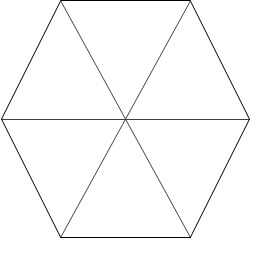You can see that there are 6 triangles formed, hence the sum of all the interior angles is (180ᣞ)(6)=1080ᣞ. Since the polygon is a regular hexagon, you know that all the interior angles are of equal size. Therefore, divide 1080ᣞ by 6 to get 120ᣞ.

## 53.

The Correct Answer is (A) — If , then and . Therefore:

\begin{align*} ab&=(x-\frac{1}{2})(x+\frac{1}{3}) \\ ab&=x^2-\frac{1}{2}x+\frac{1}{3}x-\frac{1}{6} \\ &=x^2-\frac{1}{6}x+-\frac{1}{6} \end{align*}

## 54.

The Correct Answer is (G) — Find the areas of each section. Remember to subtract the area of the inner circles when calculating the area of the outer rings. Building a table like the one below can help to keep all of your calculations organized: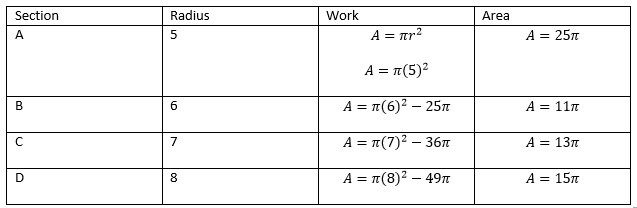Keep your answers in terms of π to avoid messy calculations. Section B has the smallest area.

## 55.

The Correct Answer is (A) — To answer this question, a diagram might help: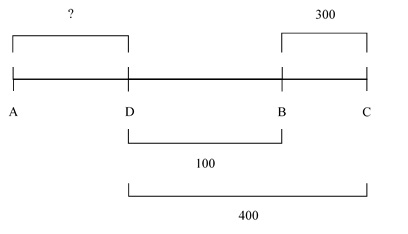On the diagram, label all the information that you know. By doing this, it is easy to see that the distance from D to B is 100 m. If his total trip was 900 m, subtract the distances that you know he travelled to find the distance between points A and D:

## 56.

The Correct Answer is (F) — To answer this question, it may help to draw a diagram: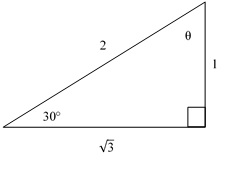You know that tan⁡θ=√3, therefore you know that the triangle will be a 30-60-90 triangle. From this, you can find that .

## 57.

The Correct Answer is (C) — Since the area of the square backyard is 64, the side length of the backyard must be . To answer this question, it may help to draw a diagram: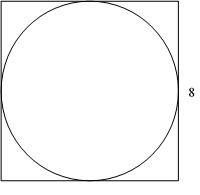If the side lengths of the square is 8 and the circular pool is perfectly inscribed in the square to create the maximum area, then the radius must be half the length of the square, or 4. Therefore, the area of a circle with radius 4 is .

## 58.

The Correct Answer is (H) — The area of the sticker is equal to the area of the cassette tape, minus the two circular holes. From the diagram, you can see that the combined diameters of the two holes is 1 in, therefore the radius of one hole must be 0.25 in. The area of both of these holes is . To find the area of the sticker, subtract the area of the holes from the area of the cassette tape.
\begin{align*} A&=(4.0)(2.5)-0.125\pi \\ A&=10-0.125\pi \\ A&\approx9.6 \end{align*}

## 59.

The Correct Answer is (A) — Any set that contains 0 can be eliminated, since that means the fraction will be undefined. Now, you are left with answer choices (A), (B), and (D). If x equals a negative number, then and the inequality is no longer true; you can eliminate answer choice (D). Realize that when x is equal to any number that is equal to or greater than 2, and the inequality is no longer true; you can eliminate answer choice (B). Therefore, (A) is the correct answer.

## 60.

The Correct Answer is (F) — From looking at the graph, you can see that there was no horizontal or vertical translations from f(x) to g(x), therefore answer choices (H) and (K) can be eliminated. The period of has not changed from f(x) to g(x), therefore answer choice J can be eliminated. The amplitude of the function has changed from f(x) to g(x), and it has increased, therefore a increased.